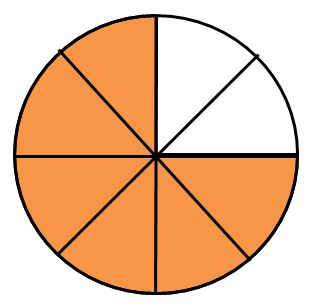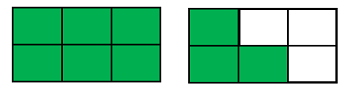# What is Fractional Notation? - Definition & Conversion

Instructions:

question 1 of 3

### What is the fractional notation for the image?Create Your Account To Take This Quiz

As a member, you'll also get unlimited access to over 79,000 lessons in math, English, science, history, and more. Plus, get practice tests, quizzes, and personalized coaching to help you succeed.

Try it risk-free for 30 days. Cancel anytime

### 1. What is the fractional notation for the image?### 2. Write the mixed number 4 1/3 in fractional notation.

Create your account to access this entire worksheet
Quizzes, practice exams & worksheets
Certificate of Completion
Create an account to get started

Test out your knowledge of fractions and fractional notation with this quiz and worksheet combo. You can find questions on the numerator or denominator of a fraction, as well as converting a mixed number into a fraction.

## Quiz & Worksheet Goals

You will need to understand the following topics to pass the quiz:

• Numerators
• Denominators
• Writing mixed numbers
• Using images to represent fractions

## Skills Practiced

• Information recall - remember what you have learned about converting mixed numbers
• Knowledge application - use what you know to answer questions about fractional notation
• Making connections - see how mixed numbers are converted into fractional notation
• Problem solving - practice solving fractional notation math problems

Head over to the lesson titled What is Fractional Notation? - Definition & Conversion to keep studying. There, you can look into the following content:

• Improper fractions
• Examples of mixed numbers
• Using graphs
Final ExamMath for Kids
Status: Not Started
Chapter ExamRepresenting Numbers for Elementary School
Status: Not Started

Support